# Perceptron

9 de May de 2010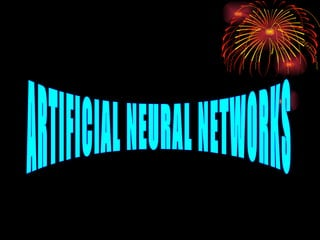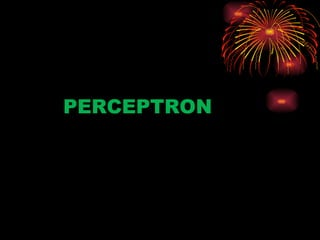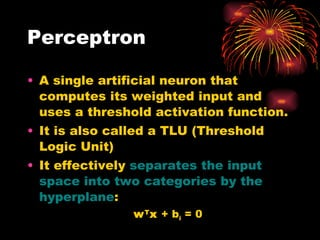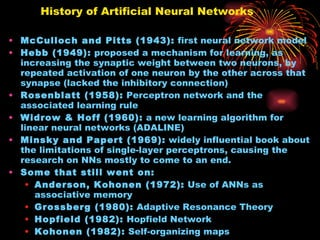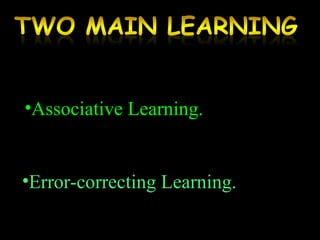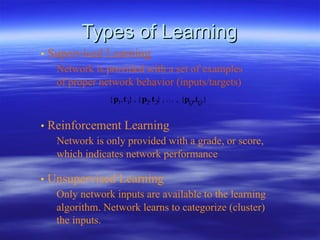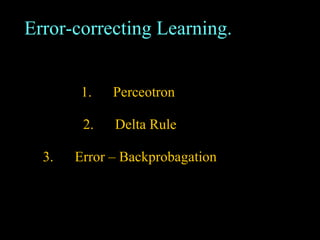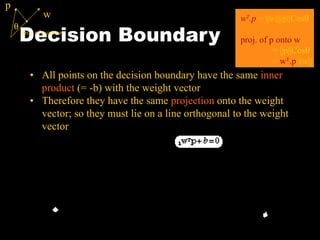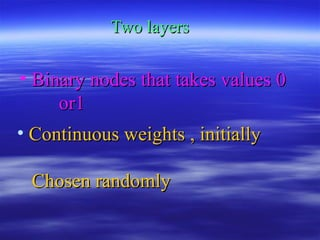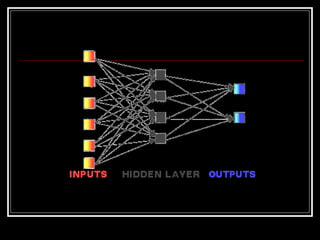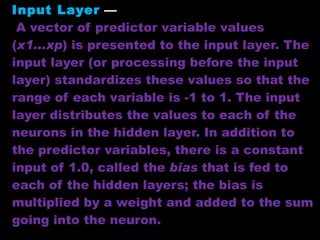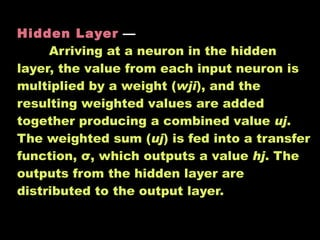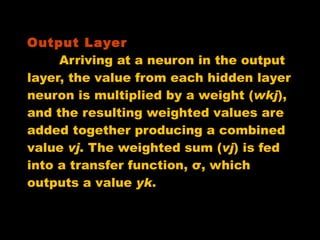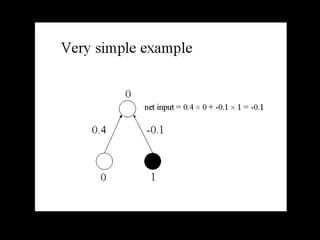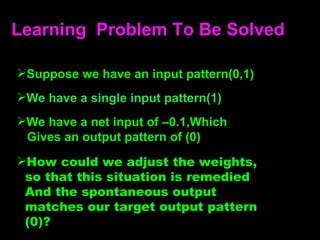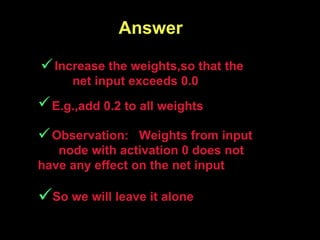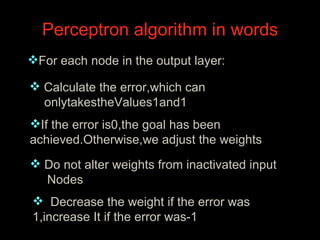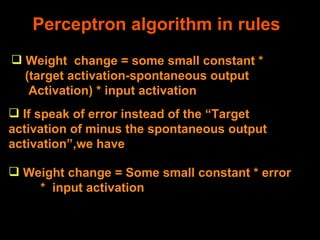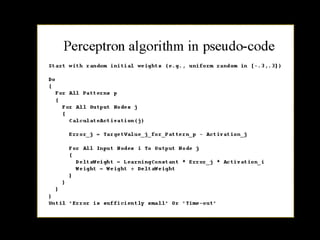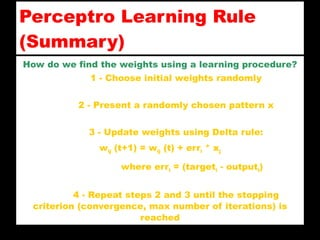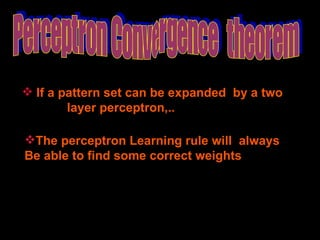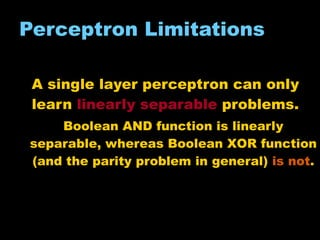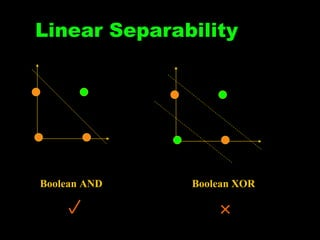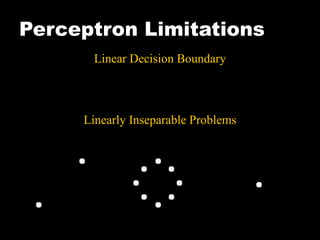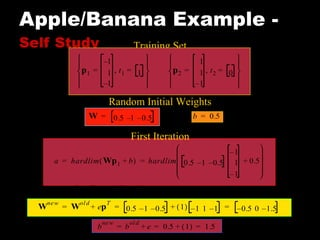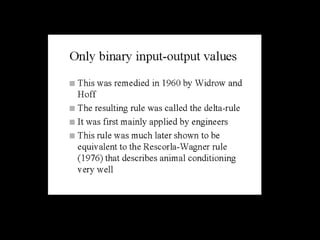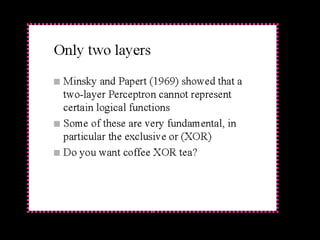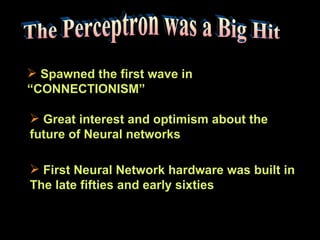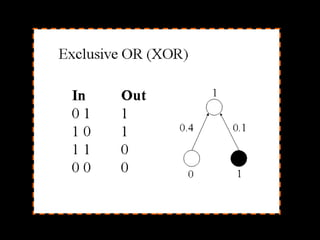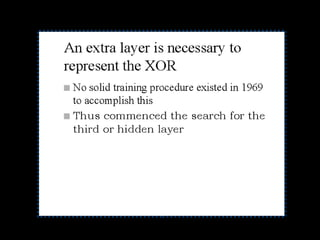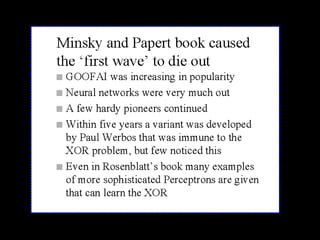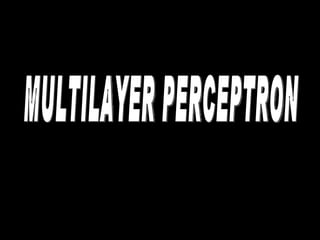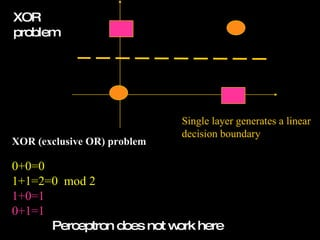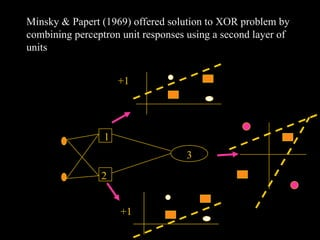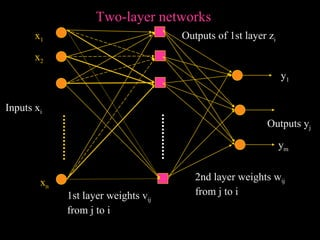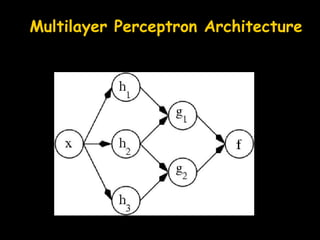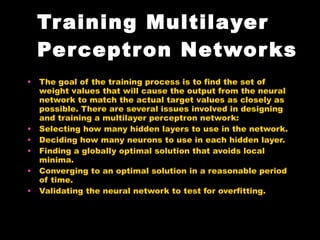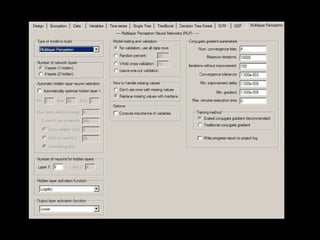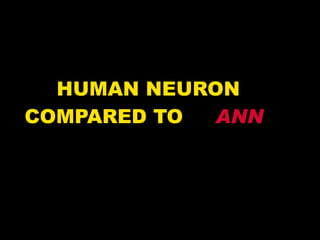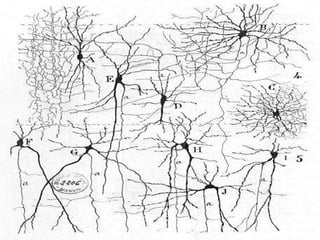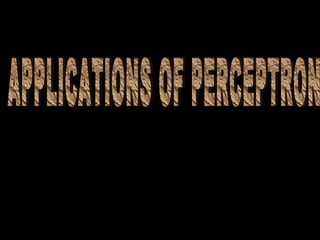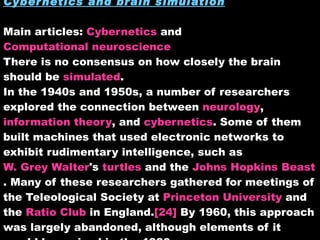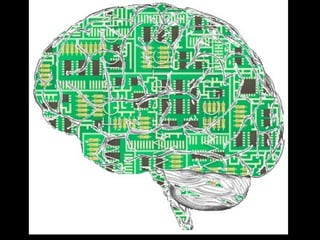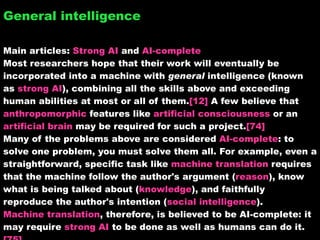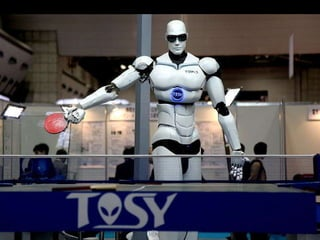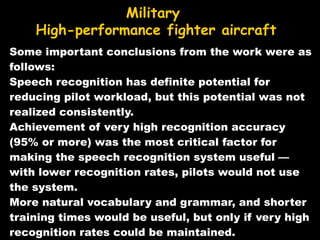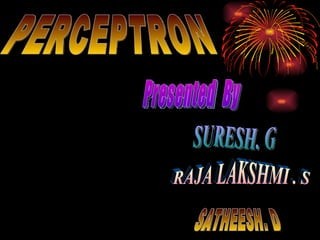1 de 49

### Perceptron

• 1.
• 4.
• 5.
• 6.
• 7. Types of Learnin g • Supervised Learning Network is provided with a set of examples of proper network behavior (inputs/targets) • Reinforcement Learning Network is only provided with a grade, or score, which indicates network performance • Unsupervised Learning Only network inputs are available to the learning algorithm. Network learns to categorize (cluster) the inputs.
• 8.
• 9. Decision Boundary • All points on the decision boundary have the same inner product (= -b) with the weight vector • Therefore they have the same projection onto the weight vector ; so they must lie on a line orthogonal to the weight vector w T .p = ||w||||p||Cos  proj. of p onto w = ||p||Cos  = w T .p /||w||  p w proj. of p onto w
• 10.
• 11.
• 12. Input Layer — A vector of predictor variable values ( x1...xp ) is presented to the input layer. The input layer (or processing before the input layer) standardizes these values so that the range of each variable is -1 to 1. The input layer distributes the values to each of the neurons in the hidden layer. In addition to the predictor variables, there is a constant input of 1.0, called the bias that is fed to each of the hidden layers; the bias is multiplied by a weight and added to the sum going into the neuron.
• 13. Hidden Layer — Arriving at a neuron in the hidden layer, the value from each input neuron is multiplied by a weight ( wji ), and the resulting weighted values are added together producing a combined value uj . The weighted sum ( uj ) is fed into a transfer function, σ, which outputs a value hj . The outputs from the hidden layer are distributed to the output layer.
• 14. Output Layer Arriving at a neuron in the output layer, the value from each hidden layer neuron is multiplied by a weight ( wkj ), and the resulting weighted values are added together producing a combined value vj . The weighted sum ( vj ) is fed into a transfer function, σ, which outputs a value yk .
• 15.
• 16.
• 17.
• 18.
• 19.
• 20.
• 21.
• 22.
• 23.
• 24. Linear Separability Boolean AND Boolean X OR
• 25. Perceptron Limitations Linear Decision Boundary Linearly Inseparable Problems
• 26. Apple/Banana Example - Self Study Training Set Random Initial Weights First Iteration e t 1 a – 1 0 – 1 = = =
• 27.
• 28.
• 29.
• 30.
• 31.
• 32.
• 34. XOR problem XOR (exclusive OR) problem 0+0=0 1+1=2=0 mod 2 1+0=1 0+1=1 Perceptron does not work here Single layer generates a linear decision boundary
• 35. Minsky & Papert (1969) offered solution to XOR problem by combining perceptron unit responses using a second layer of units 1 2 +1 3 +1
• 36. x n x 1 x 2 Inputs x i Outputs y j Two-layer networks y 1 y m 2nd layer weights w ij from j to i 1st layer weights v ij from j to i Outputs of 1st layer z i
• 38.
• 39.
• 41.
• 43. Cybernetics and brain simulation Main articles: Cybernetics and Computational neuroscience There is no consensus on how closely the brain should be simulated . In the 1940s and 1950s, a number of researchers explored the connection between neurology , information theory , and cybernetics . Some of them built machines that used electronic networks to exhibit rudimentary intelligence, such as W. Grey Walter 's turtles and the Johns Hopkins Beast . Many of these researchers gathered for meetings of the Teleological Society at Princeton University and the Ratio Club in England.  By 1960, this approach was largely abandoned, although elements of it would be revived in the 1980s.
• 44.
• 45. General intelligence Main articles: Strong AI and AI-complete Most researchers hope that their work will eventually be incorporated into a machine with general intelligence (known as strong AI ), combining all the skills above and exceeding human abilities at most or all of them.  A few believe that anthropomorphic features like artificial consciousness or an artificial brain may be required for such a project.  Many of the problems above are considered AI-complete : to solve one problem, you must solve them all. For example, even a straightforward, specific task like machine translation requires that the machine follow the author's argument ( reason ), know what is being talked about ( knowledge ), and faithfully reproduce the author's intention ( social intelligence ). Machine translation , therefore, is believed to be AI-complete: it may require strong AI to be done as well as humans can do it. 
• 46.
• 47. Some important conclusions from the work were as follows: Speech recognition has definite potential for reducing pilot workload, but this potential was not realized consistently. Achievement of very high recognition accuracy (95% or more) was the most critical factor for making the speech recognition system useful — with lower recognition rates, pilots would not use the system. More natural vocabulary and grammar, and shorter training times would be useful, but only if very high recognition rates could be maintained. Military High-performance fighter aircraft
• 48.
• 49. PERCEPTRON Presented By SURESH. G SATHEESH. D RAJA LAKSHMI . S